## 1月15日上市 曝新奥迪A6L 12款车型配置

2019年01月08日 13:59 来源：车行天下 超过：11485次关注

日前，我们从相关渠道获取到了一汽-大众奥迪全新A6L(参数|询价)车型的配置信息。新车共将推出搭载2.0T低功率/2.0T高功率/3.0T V6三种动力水平的共计12款车型，详见下表。另据悉，全新A6L将于2019年1月15日上市。

 全新A6L 车型列表 动力系统 车型名称 售价（万元） 2.0T/190马力/前驱 40TFSI 进取致雅型 待公布 40TFSI 进取动感型 2.0T/224马力/前驱 45TFSI 时尚致雅型 45TFSI 时尚动感型 2.0T/224马力/四驱 45TFSI quattro 时尚致雅型 45TFSI quattro 时尚动感型 45TFSI quattro 豪华致雅型 45TFSI quattro 豪华动感型 3.0T V6/340马力/四驱 55TFSI quattro 豪华致雅型 55TFSI quattro 豪华动感型 55TFSI quattro 尊贵致雅型 55TFSI quattro 尊贵动感型 制表：汽车之家新闻频道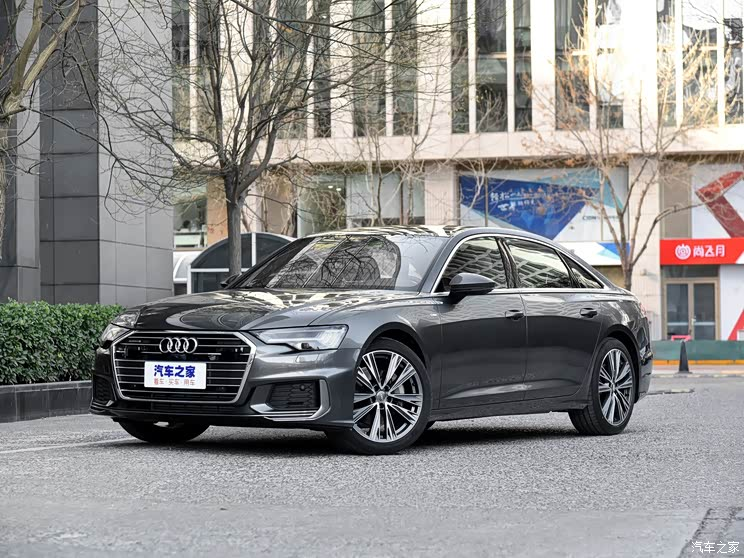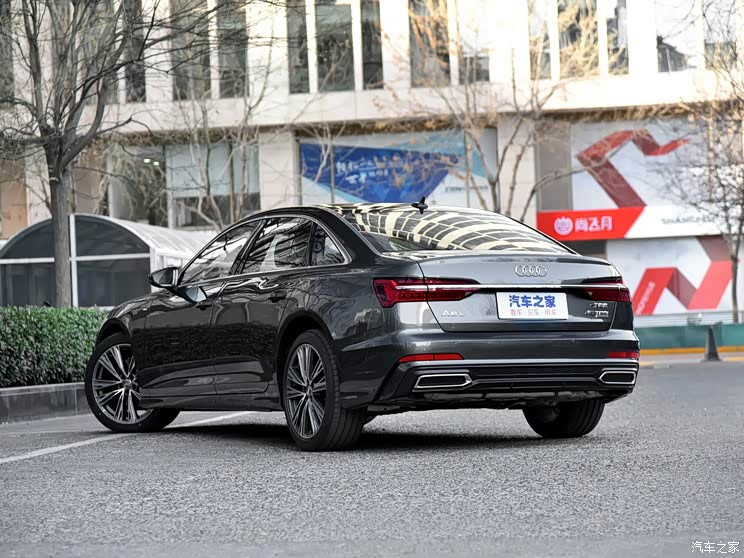『45TFSI quattro时尚动感型』

全系A6L全系标配胎压监测、非全尺寸备胎、LED大灯/尾灯（带流水转向灯和大灯清洗）、渐进式转向系统、前排座椅电动调节+电动腰托、MMI娱乐系统、奥迪虚拟座舱、定速巡航、前/侧气囊+气帘、自动启停、奥迪整体式预安全系统基础版/城市版、Audi Connect互联科技等。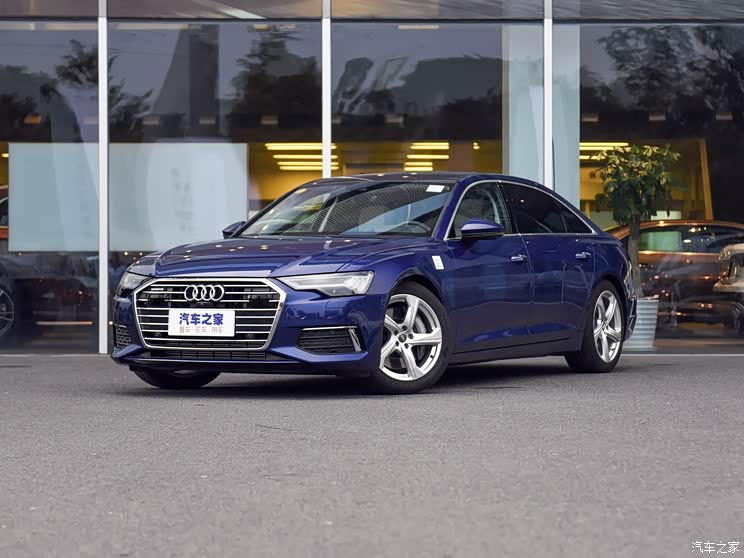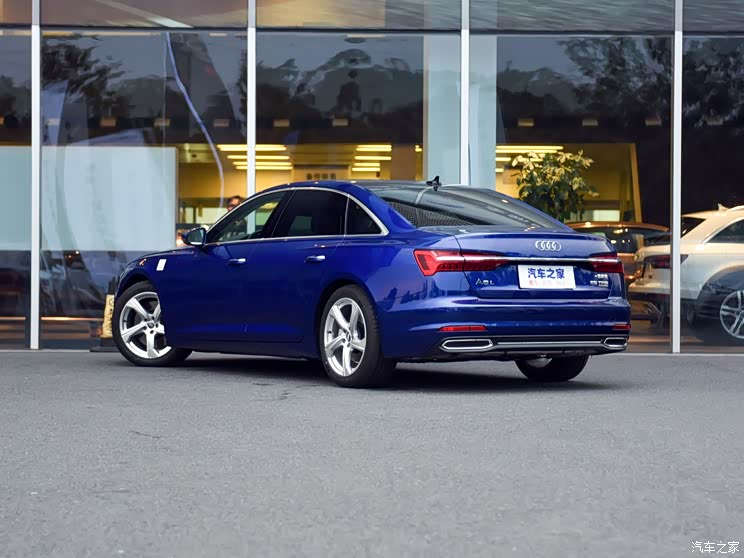『55TFSI quattro豪华致雅型』

外部配置方面，全部6款致雅型采用了常规外观方案，而6款动感型则采用S-line外观方案，同等配置下动感型的轮圈比致雅型大1英寸（顶配除外），其中两驱车型为18/19英寸，四驱车型则为19/20英寸；另外，新车标配外后视镜电动折叠功能，而3款豪华/尊贵致雅型进一步提供了自动防眩目+记忆功能（其余车型选装该配置需与舒适型座椅绑定选装）；而除了两款40TFSI车型配备了普通天窗外，其余10款车型还均配备了双天窗；前大灯方面，新车标配矩阵式LED大灯和带动态转向灯的LED尾灯，顶配的尊贵致雅/动感型则配备了高清矩阵式LED大灯和带有动态效果的尾灯。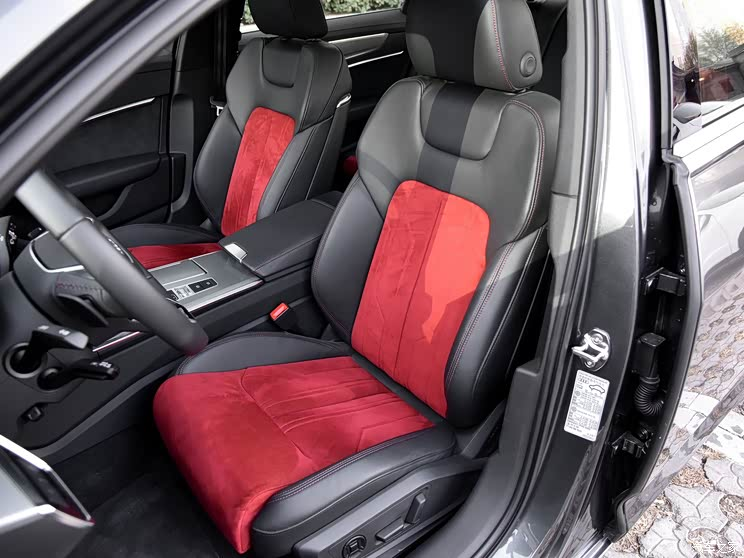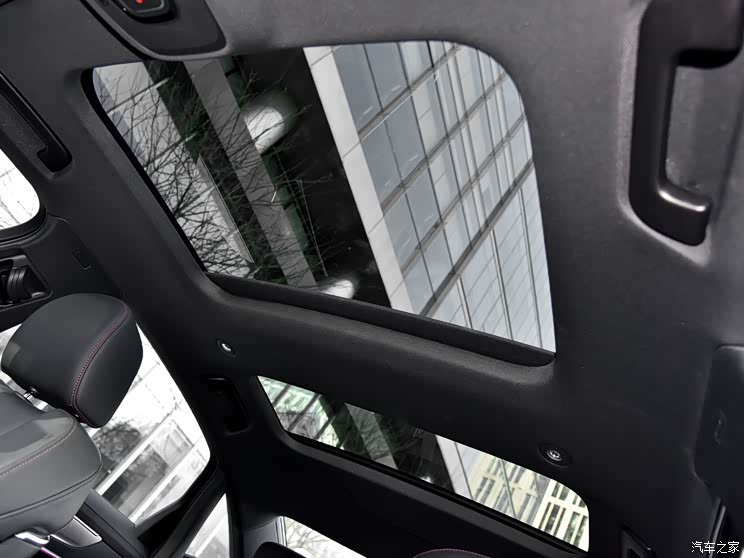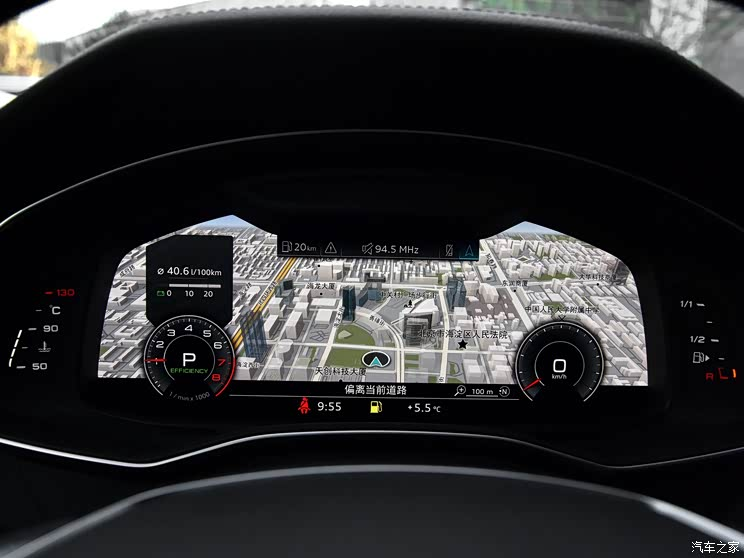『45TFSI quattro时尚动感型』

新车提供4种座椅材质，其中2.0T全部8款车型中的4款致雅型标配Milano真皮座椅，而另外4款动感型则配备了Alcantara与珠光Nappa真皮拼接包裹的运动座椅；3.0T车型则配备了Valcona真皮座椅（带后排电动调节），但该配置全系车型均可选装。此外根据车型不同，全新A6L还提供了前后排座椅通风/按摩/加热，以及样式和颜色丰富的车内饰条、普通织物/黑色织物顶棚等。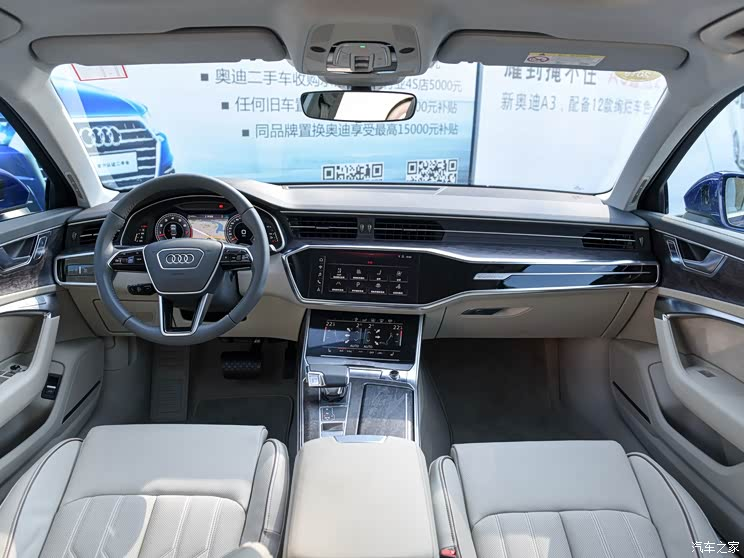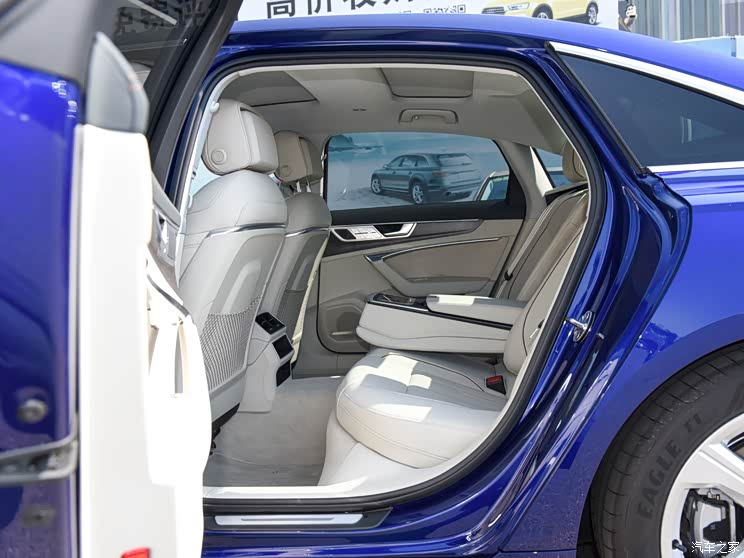『55TFSI quattro豪华致雅型』

其它车内配置方面，新车提供三辐多功能真皮方向盘（3.0T两款动感型为平底式设计），全系可选装方向盘加热；除40TFSI车型外，其余10款车型还提供了四区自动空调。此外，3.0T车型均可选装自适应空气悬架。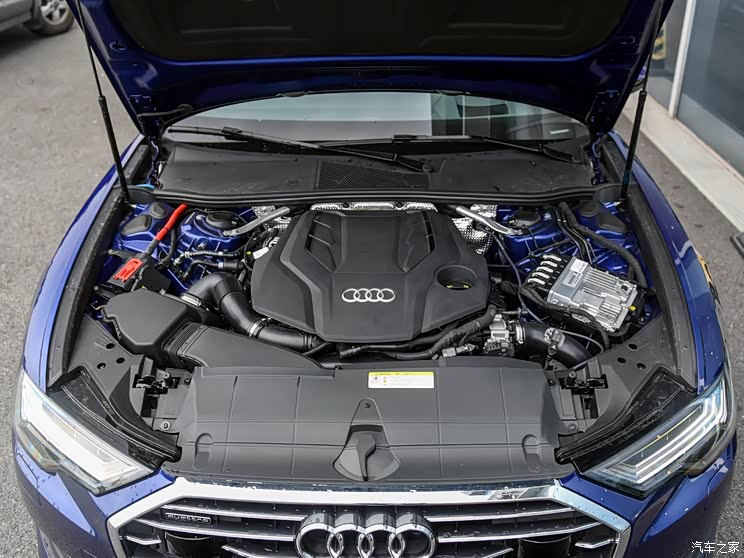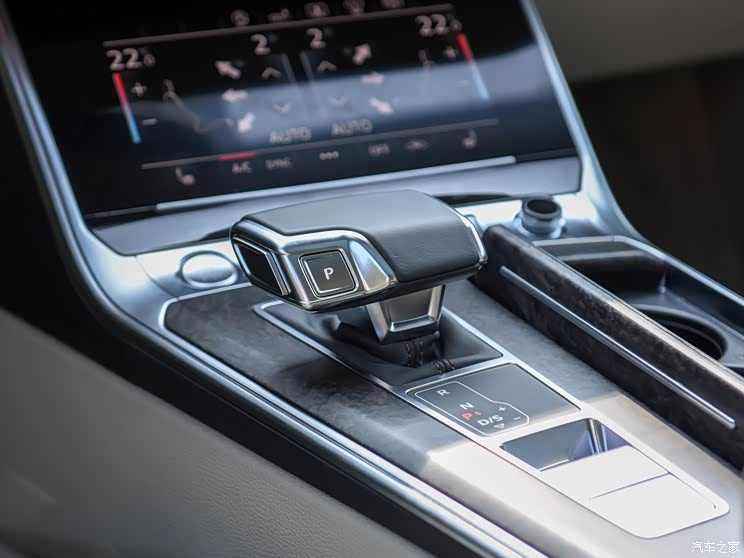『55TFSI quattro豪华致雅型』

动力系统方面，新车提供2.0T直列四缸和3.0T V6两款发动机，前者提供两种功率调校版本，匹配12V弱混系统，其中40TFSI车型最大功率190马力，峰值扭矩320牛·米；45TFSI车型为224马力/370牛·米；搭载3.0T V6发动机的55TFSI车型最大功率340马力，峰值扭矩500牛·米。传动系统方面，新车全系标配7速双离合变速箱，而45TFSI和55TFSI车型还匹配有quattro四驱系统。

#### 相关文章

0-500 字已有评论 0条 查看评论>>

### 热门标签

﻿
• 快速找车
• 选择品牌
• 选择品牌
• A  奥迪
• A  阿斯顿·马丁
• A  阿尔法·罗密欧
• B  宝沃
• B  布加迪
• B  巴博斯
• B  保时捷
• B  宾利
• B  奔驰
• B  宝马
• B  本田
• B  别克
• B  标致
• B  比亚迪
• B  宝骏
• B  北汽制造
• B  北汽新能源
• B  北汽幻速
• B  北汽威旺
• B  北京汽车
• B  奔腾
• B  北汽绅宝
• C  长安
• C  长安商用
• C  长城
• C  昌河
• D  大众
• D  道奇
• D  DS
• D  东南
• D  东风风神
• D  东风风行
• D  东风小康
• D  东风风度
• D  东风
• F  福特
• F  丰田
• F  菲亚特
• F  法拉利
• F  福田
• F  福迪
• F  福汽启腾
• G  观致
• G  广汽传祺
• G  广汽吉奥
• G  GMC
• H  红旗
• H  汉腾汽车
• H  哈弗
• H  哈飞
• H  海格
• H  海马
• H  华颂
• H  黄海
• H  华泰
• H  恒天
• J  吉利汽车
• J  捷豹
• J  Jeep
• J  江淮
• J  江铃
• J  金杯
• J  九龙
• J  金旅
• K  凯翼
• K  凯迪拉克
• K  克莱斯勒
• K  科尼塞克
• K  卡威
• K  开瑞
• L  路虎
• L  林肯
• L  劳斯莱斯
• L  兰博基尼
• L  雷克萨斯
• L  铃木
• L  雷诺
• L  理念
• L  力帆
• L  莲花汽车
• L  猎豹
• L  路特斯
• L  陆风
• M  马自达
• M  MG
• M  MINI
• M  玛莎拉蒂
• M  摩根
• M  迈凯轮
• N  纳智捷
• O  欧宝
• O  讴歌
• O  欧朗
• Q  奇瑞
• Q  起亚
• Q  启辰
• R  日产
• R  荣威
• R  瑞麒
• S  三菱
• S  斯威汽车
• S  萨博
• S  smart
• S  斯柯达
• S  斯巴鲁
• S  思铭
• S  双龙
• S  上汽大通
• S  双环
• T  特斯拉
• T  腾势
• W  沃尔沃
• W  五菱汽车
• W  五十铃
• W  威兹曼
• W  威麟
• X  现代
• X  雪佛兰
• X  雪铁龙
• X  西雅特
• Y  一汽
• Y  英菲尼迪
• Y  英致
• Y  依维柯
• Y  野马汽车
• Y  永源
• Z  众泰
• Z  中华
• Z  中兴
• Z  知豆
• 选择车系
• 选择车系
• 车型对比
• 选择品牌
• 选择品牌
• A  奥迪
• A  阿斯顿·马丁
• A  阿尔法·罗密欧
• B  宝沃
• B  布加迪
• B  巴博斯
• B  保时捷
• B  宾利
• B  奔驰
• B  宝马
• B  本田
• B  别克
• B  标致
• B  比亚迪
• B  宝骏
• B  北汽制造
• B  北汽新能源
• B  北汽幻速
• B  北汽威旺
• B  北京汽车
• B  奔腾
• B  北汽绅宝
• C  长安
• C  长安商用
• C  长城
• C  昌河
• D  大众
• D  道奇
• D  DS
• D  东南
• D  东风风神
• D  东风风行
• D  东风小康
• D  东风风度
• D  东风
• F  福特
• F  丰田
• F  菲亚特
• F  法拉利
• F  福田
• F  福迪
• F  福汽启腾
• G  观致
• G  广汽传祺
• G  广汽吉奥
• G  GMC
• H  红旗
• H  汉腾汽车
• H  哈弗
• H  哈飞
• H  海格
• H  海马
• H  华颂
• H  黄海
• H  华泰
• H  恒天
• J  吉利汽车
• J  捷豹
• J  Jeep
• J  江淮
• J  江铃
• J  金杯
• J  九龙
• J  金旅
• K  凯翼
• K  凯迪拉克
• K  克莱斯勒
• K  科尼塞克
• K  卡威
• K  开瑞
• L  路虎
• L  林肯
• L  劳斯莱斯
• L  兰博基尼
• L  雷克萨斯
• L  铃木
• L  雷诺
• L  理念
• L  力帆
• L  莲花汽车
• L  猎豹
• L  路特斯
• L  陆风
• M  马自达
• M  MG
• M  MINI
• M  玛莎拉蒂
• M  摩根
• M  迈凯轮
• N  纳智捷
• O  欧宝
• O  讴歌
• O  欧朗
• Q  奇瑞
• Q  起亚
• Q  启辰
• R  日产
• R  荣威
• R  瑞麒
• S  三菱
• S  斯威汽车
• S  萨博
• S  smart
• S  斯柯达
• S  斯巴鲁
• S  思铭
• S  双龙
• S  上汽大通
• S  双环
• T  特斯拉
• T  腾势
• W  沃尔沃
• W  五菱汽车
• W  五十铃
• W  威兹曼
• W  威麟
• X  现代
• X  雪佛兰
• X  雪铁龙
• X  西雅特
• Y  一汽
• Y  英菲尼迪
• Y  英致
• Y  依维柯
• Y  野马汽车
• Y  永源
• Z  众泰
• Z  中华
• Z  中兴
• Z  知豆
• 选择车系
• 选择车系
• 选择车型
• 选择车型
• 意见反馈